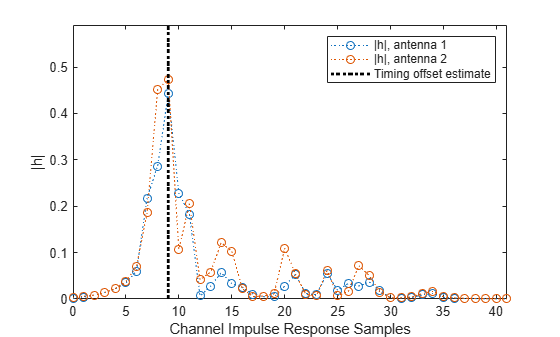# nrPerfectTimingEstimate

Perfect timing estimation

Since R2018b

## Syntax

``[offset,mag] = nrPerfectTimingEstimate(pathGains,pathFilters)``

## Description

example

````[offset,mag] = nrPerfectTimingEstimate(pathGains,pathFilters)` performs perfect timing estimation. To find the peak of the channel impulse response, the function first reconstructs the impulse response from the channel path gains `pathGains` and the path filter impulse response `pathFilters`. The channel impulse response is averaged across all channel snapshots and summed across all transmit and receive antennas before timing estimation. The function returns the estimated timing offset `offset` and the channel impulse response magnitude `mag`.```

## Examples

collapse all

Define a channel configuration structure using an `nrTDLChannel` System object. Use delay profile TDL-C from TR 38.901 Section 7.7.2.

```tdl = nrTDLChannel; tdl.DelayProfile = 'TDL-C'; tdl.DelaySpread = 100e-9;```

Create a random waveform with a duration of 1 subframe.

```tdlInfo = info(tdl); Nt = tdlInfo.NumTransmitAntennas; in = complex(zeros(100,Nt),zeros(100,Nt));```

Transmit the input waveform through the channel.

`[~,pathGains] = tdl(in);`

Obtain the path filters used in channel filtering.

`pathFilters = getPathFilters(tdl);`

Estimate timing offset.

`[offset,mag] = nrPerfectTimingEstimate(pathGains,pathFilters);`

Plot the magnitude of the channel impulse response and the timing offset estimate.

```[Nh,Nr] = size(mag); plot(0:(Nh-1),mag,'o:'); hold on; plot([offset offset],[0 max(mag(:))*1.25],'k:','LineWidth',2); axis([0 Nh-1 0 max(mag(:))*1.25]); legends = "|h|, antenna " + num2cell(1:Nr); legend([legends "Timing offset estimate"]); ylabel('|h|'); xlabel('Channel Impulse Response Samples');```## Input Arguments

collapse all

Channel path gains of the fading process, specified as an NCS-by-NP-by-NT-by-NR complex matrix, where:

• NCS is the number of channel snapshots.

• NP is the number of paths.

• NT is the number of transmit antennas.

• NR is the number of receive antennas.

Data Types: `single` | `double`
Complex Number Support: Yes

Path filter impulse response, specified as an NH-by-NP real matrix, where:

• NH is the number of impulse response samples.

• NP is the number of paths.

Each column of the matrix contains the filter impulse response for each path of the delay profile.

Data Types: `double`

## Output Arguments

collapse all

Timing offset in samples, returned as a nonnegative integer. The number of samples is relative to the first sample of the channel impulse response reconstructed from `pathGains` and `pathFilters`.

Data Types: `double`

Channel impulse response magnitude for each receive antenna, returned as an NH-by-NR real matrix.

• NH is the number of impulse response samples.

• NR is the number of receive antennas.

`mag` inherits its data type from `pathGains`.

Data Types: `single` | `double`

## Version History

Introduced in R2018b